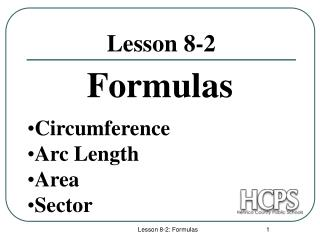# Lesson 8-2 - PowerPoint PPT PresentationDownload PresentationLesson 8-2

Lesson 8-2Download Presentation## Lesson 8-2

- - - - - - - - - - - - - - - - - - - - - - - - - - - E N D - - - - - - - - - - - - - - - - - - - - - - - - - - -
##### Presentation Transcript

1. Formulas Lesson 8-2 • Circumference • Arc Length • Area • Sector Lesson 8-2: Formulas

2. Central Angle A central angle is an angle whose vertex is at the center of the circle. The arc measure is written as The measure of a minor arc is the measure of its central angle. The measure of a major arc is 360 minus the measure of its central angle. Lesson 8-2: Formulas

3. 3 cm CIRCUMFERENCE: Circumference is the distance around the circle. Formula: Or where Example: Find the circumference of the following circle. cm Lesson 8-2: Formulas

4. A ° 72 B 2 cm C Arc Length Arc length is the distance around an arc. Formula: The circumference multiplied by the ratio of the center angle and 360°. Example: Arc Length Lesson 8-2: Formulas

5. 3 cm Area of a Circle Area of a circle is the number of unit squares that can fit into a circle. Formula: Example: Find the area of the following circle. Lesson 8-2: Formulas

6. Area of a Sector Area of a sector is the area of a section of the circle. Formula: The area multiplied by the ratio of the center angle and 360° Example: Sector 65° Lesson 8-2: Formulas